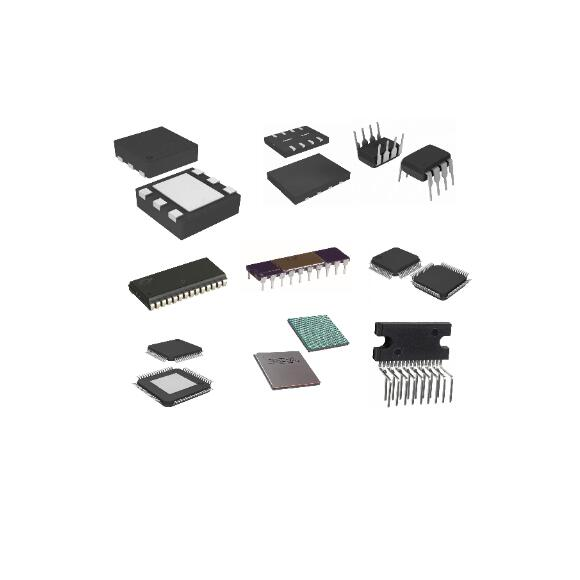< img src="https://mc.yandex.ru/watch/53493796" style="position:absolute; left:-9999px;" alt="" />
Need Help?• Type: integrated circuit
• Brand Name: Brand new
• Package: 16-SOIC, Tube
• Voltage – Breakdown: Standard
• Frequency – Switching: Standard
• Power (Watts): Standard
• Operating Temperature: Standard
• Mounting Type: Standard
• Voltage – Supply (Min): Standard
• Voltage – Supply (Max): Standard
• Voltage – Output: Standard
• Current – Output / Channel: Standard
• Frequency: Standard
• Applications: Standard
• FET Type: Standard
• Voltage – Supply: Standard
• Frequency – Max: Standard
• Power – Max: Standard
• Tolerance: Standard
• Function: Standard
• Voltage Supply – Internal: Standard
• Frequency – Cutoff or Center: Standard
• Current – Leakage (IS(off)) (Max): Standard
• Isolated Power: Standard
• Voltage – Isolation: Standard
• Current – Output High, Low: Standard
• Current – Peak Output: Standard
• Voltage – Forward (Vf) (Typ): Standard
• Current – DC Forward (If) (Max): Standard
• Input Type: Standard
• Output Type: Standard
• Current Transfer Ratio (Min): Standard
• Current Transfer Ratio (Max): Standard
• Voltage – Output (Max): Standard
• Voltage – Off State: Standard
• Static dV/dt (Min): Standard
• Current – LED Trigger (Ift) (Max): Standard
• Current – On State (It (RMS)) (Max): Standard
• Impedance: Standard
• Impedance – Unbalanced/Balanced: Standard
• LO Frequency: Standard
• RF Frequency: Standard
• Input Range: Standard
• Output Power: Standard
• Frequency Bands (Low / High): Standard
• Specifications: Standard
• Size / Dimension: Standard
• Modulation or Protocol: Standard
• Interface: Standard
• Power – Output: Standard
• Memory Size: Standard

## Description

• Type: integrated circuit
• Brand Name: Brand new
• Package: 16-SOIC, Tube
• Voltage – Breakdown: Standard
• Frequency – Switching: Standard
• Power (Watts): Standard
• Operating Temperature: Standard
• Mounting Type: Standard
• Voltage – Supply (Min): Standard
• Voltage – Supply (Max): Standard
• Voltage – Output: Standard
• Current – Output / Channel: Standard
• Frequency: Standard
• Applications: Standard
• FET Type: Standard
• Current – Output (Max): Standard
• Current – Supply: Standard
• Voltage – Supply: Standard
• Frequency – Max: Standard
• Power – Max: Standard
• Tolerance: Standard
• Function: Standard
• Voltage Supply – Internal: Standard
• Frequency – Cutoff or Center: Standard
• Current – Leakage (IS(off)) (Max): Standard
• Isolated Power: Standard
• Voltage – Isolation: Standard
• Current – Output High, Low: Standard
• Current – Peak Output: Standard
• Voltage – Forward (Vf) (Typ): Standard
• Current – DC Forward (If) (Max): Standard
• Input Type: Standard
• Output Type: Standard
• Current Transfer Ratio (Min): Standard
• Current Transfer Ratio (Max): Standard
• Voltage – Output (Max): Standard
• Voltage – Off State: Standard
• Static dV/dt (Min): Standard
• Current – LED Trigger (Ift) (Max): Standard
• Current – On State (It (RMS)) (Max): Standard
• Impedance: Standard
• Impedance – Unbalanced/Balanced: Standard
• LO Frequency: Standard
• RF Frequency: Standard
• Input Range: Standard
• Output Power: Standard
• Frequency Bands (Low / High): Standard
• Specifications: Standard
• Size / Dimension: Standard
• Modulation or Protocol: Standard
• Interface: Standard
• Power – Output: Standard
• Memory Size: Standard
• Protocol: Standard
• Modulation: Standard
• Serial Interfaces: Standard
• GPIO: Standard
• Utilized IC / Part: Standard
• Standards: Standard

SEND EMAIL TO US